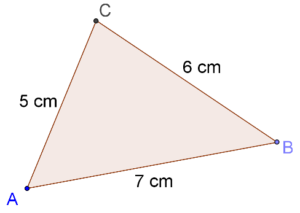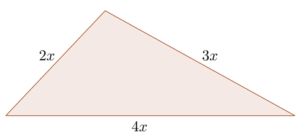# Heron's Formula

Go back to  'Triangles-Quadrilaterals'

Consider $$\Delta ABC$$ as shown below:How will you find the area of this triangle? Assuming you were to take any one of the three sides as the base, how would you find the altitude? Reflect on this problem for a moment?

From this problem, it should be clear that it might not always be possible to directly apply the simple formula for the area of a triangle (½ × base × height). How do we calculate the area of a triangle in such a case?

Heron’s Formula helps us in such situations. To understand this formula, we first defined something called the semi-perimeter. The semi-perimeter is simply half of the perimeter of a triangle, and is generally denoted by [ms]. In the example above, the semi-perimeter is equal to:

$s = \frac{{5 + 6 + 7}}{2} = \frac{{18}}{2} = 9{\rm{ cm}}$

In general, for a triangle with sides a, b and c, the semi-perimeter will be given by:

$s = \frac{{a + b + c}}{2}$

Now, Heron’s Formula tells us that the area of a triangle with sides a, b and c is given by:

$A = \sqrt {s\left( {s - a} \right)\left( {s - b} \right)\left( {s - c} \right)}$

For example, for the triangle $$\Delta ABC$$ above, the area will be:

\begin{align}&A = \sqrt {s\left( {s - a} \right)\left( {s - b} \right)\left( {s - c} \right)} \\\,\,\,\,\, &\;\;\;= \sqrt {9\left( {9 - 5} \right)\left( {9 - 6} \right)\left( {9 - 7} \right)} \\\,\,\,\,\,& \;\;\;= \sqrt {9 \times 4 \times 3 \times 2} \\\,\,\,\,\, &\;\;\;= 6\sqrt 6 {\rm{ c}}{{\rm{m}}^2}\end{align}

Example 1: The semi-perimeter of $$\Delta ABC$$ is 16 cm, and AB = 8 cm and BC = 11cm. What is the area of $$\Delta ABC$$?

Solution: Since the semi-perimeter is 16 cm, the perimeter is 32 cm. Hence,

AB + BC + AC = 32

è 8 + 11 + AC = 32

è AC = 13 cm

By Heron’s Formula, the area of $$\Delta ABC$$ will be:

\begin{align}&A = \sqrt {s\left( {s - a} \right)\left( {s - b} \right)\left( {s - c} \right)} \\\,\,\,\,\,& \;\;\;= \sqrt {16\left( {16 - 8} \right)\left( {16 - 11} \right)\left( {16 - 13} \right)} \\\,\,\,\,\,& \;\;\;= \sqrt {16 \times 8 \times 5 \times 3} \\\,\,\,\,\, &\;\;\;= 8\sqrt {30} \,{\rm{c}}{{\rm{m}}^2}\end{align}

Example 2: The sides of a triangle are in the ratio 2 : 3 : 4, and its semi-perimeter is 18 cm. What is the area of the triangle?

Solution: We can assume the triangle’s sides to be:

$a = 2x,\,\,b = 3x,\,\,c = 4x$

Consider the following figure:Now, the semi-perimeter is given. Thus, we have:

\begin{align}&s = \frac{{a + b + c}}{2} = \frac{{2x + 3x + 4x}}{2} = \frac{{9x}}{2} = 18\\ &\Rightarrow \,\,\,x = 4\\ &\Rightarrow \,\,\,a = 8,\,\,\,b = 12,\,\,\,c = 16\end{align}

Finally, the area of this triangle can be written using Heron’s Formula:

\begin{align}&A = \sqrt {18\left( {18 - 8} \right)\left( {18 - 12} \right)\left( {18 - 16} \right)} \\&\,\,\,\,\, = \sqrt {18 \times 10 \times 6 \times 2} \\&\,\,\,\,\, = 12\sqrt {15} \,{\rm{c}}{{\rm{m}}^2}\end{align}

Example 3: The sides of a triangle are in the ratio 12 : 17 : 25, and its perimeter is 540 cm. What is its area?

Solution: We can assume the sides of the triangle to have the following lengths:

$$12x,\;17x,\;25x$$

Thus,

$\begin{array}{l}12x + 17x + 25x = 540\\ \Rightarrow \qquad 54x = 540\\ \Rightarrow \qquad x = 10\end{array}$

The lengths of the sides and the semi-perimeter are:

\begin{align}&a = 12 \times 10 = 120\;{\rm{cm}}\\&b = 17 \times 10 = 170\;{\rm{cm}}\\&c = 25 \times 10 = 250\;{\rm{cm}}\\&s = \frac{{540}}{2}\, = 270\;{\rm{cm}}\end{align}

By Heron’s Formula, the area of the triangle is:

\begin{align}&A = \sqrt {s\left( {s - a} \right)\left( {s - b} \right)\left( {s - c} \right)} \\ \;\;\; &\;\;\;= \sqrt{ 270 \times \left( {270 - 120} \right) \times \left( {270 - 170} \right)\\ \times \left( {270 - 250} \right)} \\ &\;\;\;= \sqrt {270 \times 150 \times 100 \times 20} \\& \;\;\; = \sqrt {81000000} \\& \;\;\; = 9000\;{\rm{c}}{{\rm{m}}^2}\\& \;\;\; = 0.9\;{{\rm{m}}^2} \end{align}

Example 4: Using Heron’s formula, determine the area of an equilateral triangle in terms of the length of its side(s).

Solution: Let each side of an equilateral triangle be of length x units. The semi-perimeter will then be:

$s = \frac{{x + x + x}}{2} = \frac{{3x}}{2}$

Now, applying Heron’s formula, the area of the triangle will be:

\begin{align}&A = \sqrt {s\left( {s - a} \right)\left( {s - b} \right)\left( {s - c} \right)} \\&\;\;\; = \sqrt {\frac{{3x}}{2} \times \left( {\frac{{3x}}{2} - x} \right) \times \left( {\frac{{3x}}{2} - x} \right) \times \left( {\frac{{3x}}{2} - x} \right)} \\&\;\;\; = \sqrt {\frac{{3x}}{2} \times \frac{x}{2} \times \frac{x}{2} \times \frac{x}{2}} \\&\;\;\; = \sqrt {\frac{{3{x^4}}}{{16}}} \\&\;\;\; = \frac{{\sqrt 3 \,{x^2}}}{4}\;{\rm{sq}}{\rm{.}}\;{\rm{units}}\end{align}

It is a good idea to remember this relation for the area of any equilateral triangle:

$A = \frac{{\sqrt 3 }}{4} \times {\left( {{\rm{side}}} \right)^2}$